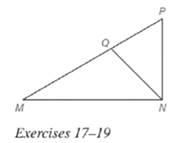Chapter 5.6, Problem 19EElementary Geometry For College St...

7th Edition
Alexander + 2 others
ISBN: 9781337614085

Solutions

Chapter
SectionElementary Geometry For College St...

7th Edition
Alexander + 2 others
ISBN: 9781337614085
Textbook Problem

Exercises 18 and 19 are based on a theorem ( not stated) that is the converse of Theorem 5.6.3. See the figure on page 267. Given: N P = 6 , M N = 9 , P Q = 4 , and M Q = 6 ; m ∠ P = 62 ∘ and m ∠ M = 36 ∘ Find: m ∠ Q N M (HINT: N P M N = P Q M Q . )To determine

To find:

mQNM.

Explanation

Given:

NQ bisects MNP, NP=6,MN=9,PQ=4,MQ=6,mP=62,mM=36.

Theorem used:

Angle bisector theorem:

If a ray bisects one angle of a triangle, then it divides the opposite side into segments whose lengths are proportional to the lengths of the two sides that form the bisected angle.

Calculation:

Given: NQ bisects MNP.

Then by angle-bisector theorem, the ray NQ divides the opposite side into segments whose lengths are proportional to the lengths of the two sides that form the bisected angle.

NPMN=PQMQ

In a triangle the sum of the angles is 180.

Still sussing out bartleby?

Check out a sample textbook solution.

See a sample solution

The Solution to Your Study Problems

Bartleby provides explanations to thousands of textbook problems written by our experts, many with advanced degrees!

Get Started

Fill in each blank: 168ft=in.

Elementary Technical Mathematics

Evaluate the integrals in Problems 1-32. 17.

Mathematical Applications for the Management, Life, and Social Sciences

Write the following numbers in word form.

Contemporary Mathematics for Business & Consumers

True or False: The absolute extrema of a continuous function on a closed interval always exist.

Study Guide for Stewart's Single Variable Calculus: Early Transcendentals, 8th